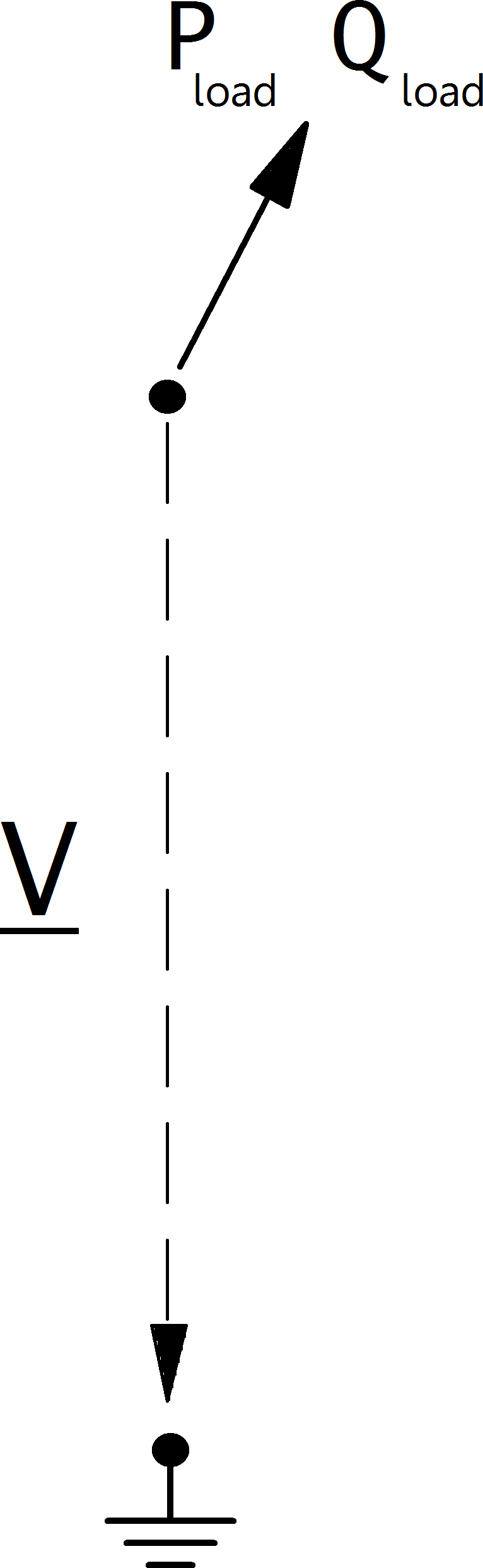## Create Function¶

pandapower.create_load(net, bus, p_kw, q_kvar=0, const_z_percent=0, const_i_percent=0, sn_kva=nan, name=None, scaling=1., index=None, in_service=True, type=None, max_p_kw=nan, min_p_kw=nan, max_q_kvar=nan, min_q_kvar=nan, controllable=nan)

All loads are modelled in the consumer system, meaning load is positive and generation is negative active power. Please pay attention to the correct signing of the reactive power as well.

INPUT:

net - The net within this load should be created

bus (int) - The bus id to which the load is connected

OPTIONAL:

p_kw (float, default 0) - The real power of the load

• negative value -> generation

q_kvar (float, default 0) - The reactive power of the load

const_z_percent (float, default 0) - percentage of p_kw and q_kvar that will be associated to constant impedance load at rated voltage

const_i_percent (float, default 0) - percentage of p_kw and q_kvar that will be associated to constant current load at rated voltage

sn_kva (float, default None) - Nominal power of the load

name (string, default None) - The name for this load

scaling (float, default 1.) - An OPTIONAL scaling factor to be set customly

type (string, None) - type variable to classify the load

index (int, None) - Force a specified ID if it is available. If None, the index one higher than the highest already existing index is selected.

in_service (boolean) - True for in_service or False for out of service

max_p_kw (float, default NaN) - Maximum active power load - necessary for controllable loads in for OPF

min_p_kw (float, default NaN) - Minimum active power load - necessary for controllable loads in for OPF

max_q_kvar (float, default NaN) - Maximum reactive power load - necessary for controllable loads in for OPF

min_q_kvar (float, default NaN) - Minimum reactive power load - necessary for controllable loads in OPF

controllable (boolean, default NaN) - States, whether a load is controllable or not. Only respected for OPF

OUTPUT:
index (int) - The unique ID of the created element
EXAMPLE:
pandapower.create_load_from_cosphi(net, bus, sn_kva, cos_phi, mode, **kwargs)

Creates a load element from rated power and power factor cos(phi).

INPUT:

net - The net within this static generator should be created

bus (int) - The bus id to which the load is connected

sn_kva (float) - rated power of the load

cos_phi (float) - power factor cos_phi

mode (str) - “ind” for inductive or “cap” for capacitive behaviour

**kwargs are passed on to the create_load function

OUTPUT:
index (int) - The unique ID of the created load

All elements are modeled from a consumer point of view. Active power will therefore always be positive, reactive power will be negative for inductive behaviour and positive for capacitive behaviour.

## Input Parameters¶

 Parameter Datatype Value Range Explanation name string name of the load bus * integer index of connected bus p_kw* float $$\geq 0$$ active power of the load [kW] q_kvar* float reactive power of the load [kVar] const_z_percent* float $$[0,100]$$ percentage of p_kw and q_kvar that is associated to constant impedance load at rated voltage [$$\%$$] const_i_percent* float $$[0,100]$$ percentage of p_kw and q_kvar that is associated to constant current load at rated voltage [$$\%$$] sn_kva float $$>0$$ rated power of the load [kVA] scaling * float $$\geq 0$$ scaling factor for active and reactive power in_service* boolean True / False specifies if the load is in service. controllable** bool States if load is controllable or not, load will not be used as a flexibilty if it is not controllable max_p_kw** float Maximum active power min_p_kw** float Minimum active power max_q_kvar** float Maximum reactive power min_q_kvar** float Minimum reactive power

*necessary for executing a power flow calculation.

Note

Loads should always have a positive p_kw value, since all power values are given in the consumer system. If you want to model constant generation, use a Static Generator (sgen element) instead of a negative load.

Note

The apparent power value sn_kva is provided as additional information for usage in controller or other applications based on panadapower. It is not considered in the power flow!

## Electric Model¶

Loads are modelled as PQ-buses in the power flow calculation, with an option to use the so-called ZIP load model, where a load is represented as a composition of constant power (P), constant current (I) and constant impedance (Z):What part of the load is considered constant with constant power, constant current or constant impedance is defined as follows:

\begin{align*} z_{const} =& const\_z\_percent / 100 \\ i_{const} =& const\_i\_percent / 100 \\ p_{const} =& (100 - const\_z\_percent - const\_i\_percent) / 100 \end{align*}

The load power values are then defines as:

\begin{align*} P_{load} =& p\_kw \cdot scaling \cdot (p_{const} + z_{const} \cdot V^2 + i_{const} \cdot V ) \\ Q_{load} =& q\_kvar \cdot scaling \cdot (p_{const} + z_{const} \cdot V^2 + i_{const} \cdot V) \end{align*}

## Result Parameters¶

The power values in the net.res_load table are equivalent to $$P_{load}$$ and $$Q_{load}$$.